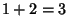## 3

3 is the only Integer which is the sum of the preceding Positive Integers () and the only number which is the sum of the Factorials of the preceding Positive Integers (). It is also the first Odd Prime. A quantity taken to the Power 3 is said to be Cubed.

See also 1, 2, 3x+1 Mapping, Cubed, Period Three Theorem, Super-3 Number, Ternary, Three-Colorable, Zero